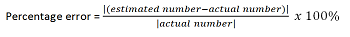# What is Percentage Error? - Definition & Example

An error occurred trying to load this video.

Try refreshing the page, or contact customer support.

Coming up next:

### You're on a roll. Keep up the good work!

Replay
Your next lesson will play in 10 seconds
• 0:00 What Is Percentage Error?
• 1:50 Application
• 2:34 Calculations
• 3:25 Lesson Summary
Save Save

Want to watch this again later?

Timeline
Autoplay
Autoplay
Speed Speed Audio mode

#### Recommended Lessons and Courses for You

Lesson Transcript
Instructor
Nola Bridgens

Nola has taught elementary school and tutored for four years. She has a bachelor's degree in Elementary Education, a master's degree in Marketing, and is a certified teacher.

Expert Contributor
Matthew Bergstresser

Matthew has a Master of Arts degree in Physics Education. He has taught high school chemistry and physics for 14 years.

In this lesson, we'll define what percentage error is, why it is useful, and how to calculate it. We'll also look at some examples of when you could use percentage error.

## What Is Percentage Error?

Look closely at the image of the gumball machine and make your best estimated guess at how many gumballs you think there are.Have you ever played this game at school or at a carnival? The person who guesses closest to the actual number of gumballs wins a prize. We're going to explore how to calculate the winner by learning about percentage error.

Percentage error is the difference between the estimated number and the actual number when compared to the actual number expressed in percent format. The formula looks like this:In other words, you take the difference between the real answer and the guessed answer, divide it by the real answer, and then turn it into a percent.

For example, let's say you guessed that there were 230 gumballs in the image, but there were actually 311 gumballs. The difference between your guess (230) and the actual number (311) in comparison to the actual number (311) expressed as a percent is the percentage error.

Did you notice the straight lines that were part of our formula? They indicate that you take the absolute value of that number, or the distance of a number from zero, without regard for negative signs. This simply means that if you get a negative number inside the absolute value signs, you treat it as if it were positive.

To calculate percentage error, you subtract the actual number from the estimated number to find the error. Then, you divide the error in absolute value by the actual number in absolute value. This gives you the error in a decimal format. From there, you can multiply by 100% to find the percentage error.

To unlock this lesson you must be a Study.com Member.

## Percentage Error

Percentage error is a way to analyze how successful an experiment was. It is used in many physical sciences including chemistry and physics experiments. Let's work on some scenarios where we can practice using the percentage error equation.

### Problems

1. A student is measuring the amount of water in a graduated cylinder and reports a value of 24 mL. The teacher reports the value as 24.2 mL. What is the student's percent error?

2. Another student was experimentally determining the boiling point of water. They reported it to be 99.5° C. The accepted value for the boiling point of water is 100° C. What is the percent error?

3. The percent error of a student's measurement of the density of aluminum is -4.2%. The accepted value of the density of aluminum is 2.7 g/cm3. What is the student's value for the density of aluminum?

### Solutions

The percentage error formula is % error = (|experimental - accepted|) / (accepted) x 100.

1. (|24 - 24.2|) / 24.2) x 100 = 0.83% error

2. (|99.5 - 100|) / 100 x 100 = 0.5% error

3. -0.042 = (x - 2.7) / 2.7

-0.1134 = x - 2.7

2.59 g/cm3 = x

### Register to view this lesson

Are you a student or a teacher?

#### See for yourself why 30 million people use Study.com

##### Become a Study.com member and start learning now.
Back
What teachers are saying about Study.com

### Earning College Credit

Did you know… We have over 200 college courses that prepare you to earn credit by exam that is accepted by over 1,500 colleges and universities. You can test out of the first two years of college and save thousands off your degree. Anyone can earn credit-by-exam regardless of age or education level.